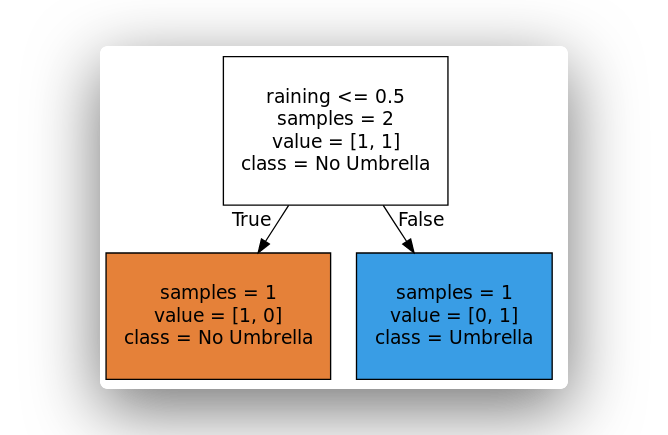Decision Trees are one of the most popular supervised machine learning algorithms.

Is a predictive model to go from observation to conclusion. Observations are represented in branches and conclusions are represented in leaves.

If the model has target variable that can take a discrete set of values, is a classification tree.

If the model has target variable that can take continuous values, is a regression tree.

Related course: Python Machine Learning Course

Decision Trees are also common in statistics and data mining. It’s a simple but useful machine learning structure.

## Decision Tree

### Introduction

How to understand Decision Trees? Let’s set a binary example!

In computer science, trees grow up upside down, from the top to the bottom.

The top item is the question called root nodes. Just like the real trees, everything starts there.

That question has two possible answers, so answers are (in this case) two branches nodes leading out of the tree.

Everything that is not a root or a branch is a leaf. Leaf nodes can be filled with another answer or criteria. Leaves can also be called decisions.

You can repeat the process till Decision Tree is complete. In theory, it’s that easy.### The Algorithm

The algorithms process it as:

A decision trees has object and objects has statements.
Each statement has features.
Features are attributes of an object.
Algorithms study this process till every statements and every feature is complete.

To use Decision Trees in a programming language the steps are:

1. Present a dataset.
2. Train a model, learning from descriptive features and a target feature.
3. Continue the tree until accomplish a criteria.
4. Create leaf nodes representing the predictions.
5. Show instances and run down the tree until arrive at leaf nodes.

Done!

### Dataset

raining decision
no no umbrella
yes take umbrella

Can be simplified as:

raining decision
0 0
1 1

So corresponding X (features) and Y (decision/label) are:

### Decision Tree code

Sklearn supporst a decision tree out of the box.
You can then run this code:

This will create the tree and output a dot file. You can use Webgraphviz to visualize the tree, by pasting the dot code in there.

The create model will be able to make predictions for unknown instances because it models the relationship between the known descriptive features and the know target feature.

### Important Concepts

Finally, quickly review 4 important concepts of Decision Trees and Machine Learning.

1. Expected Value: means the expected value of a random variable. Expected Value Analysis is made to Decision Trees to determinate severity in risks. To do it, we must measure the probability of the risk in numbers between 0.0 and 1.0.

2. Entropy: to measure the information. Is the expected amount of information that would be needed to specify whether a new instance should be classified as one or another. The idea of entropy is to quantify the uncertainty of the probability distribution with respect to the possible classification classes.

3. Accuracy: is the number of correct predictions made divided by the total number of predictions made. What we want to do is to check how accurate a machine learning model is.

4. Overfitting: happens because the training model is trying to fit as well as possible over the training data. To prevent it try to reduce the noise in your data.

That will be all for the basics of Decision Trees and Machine Learning!

If you are new to Machine Learning, then I highly recommend this book.